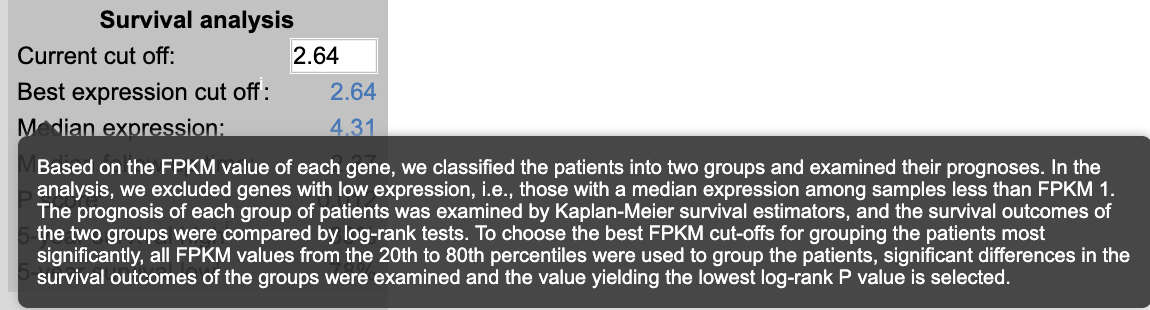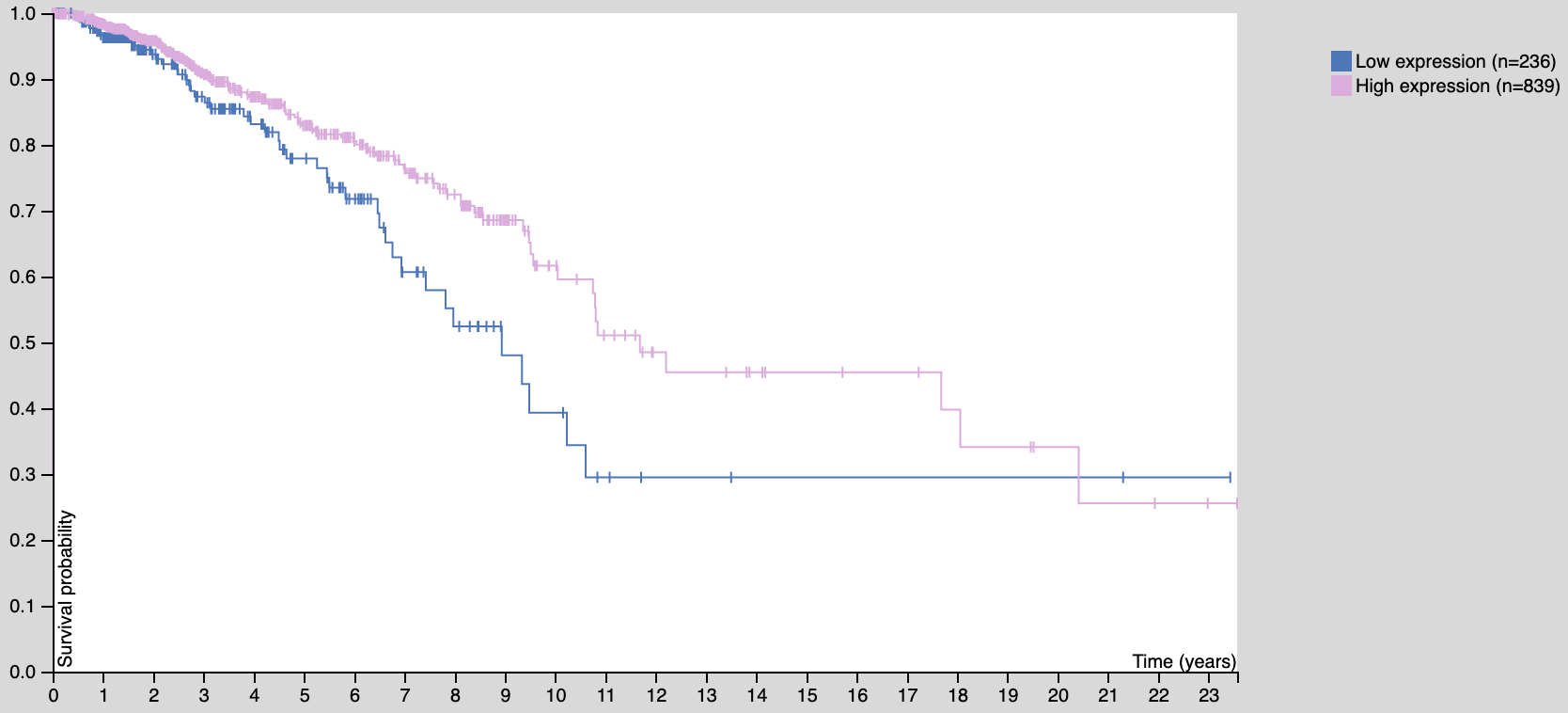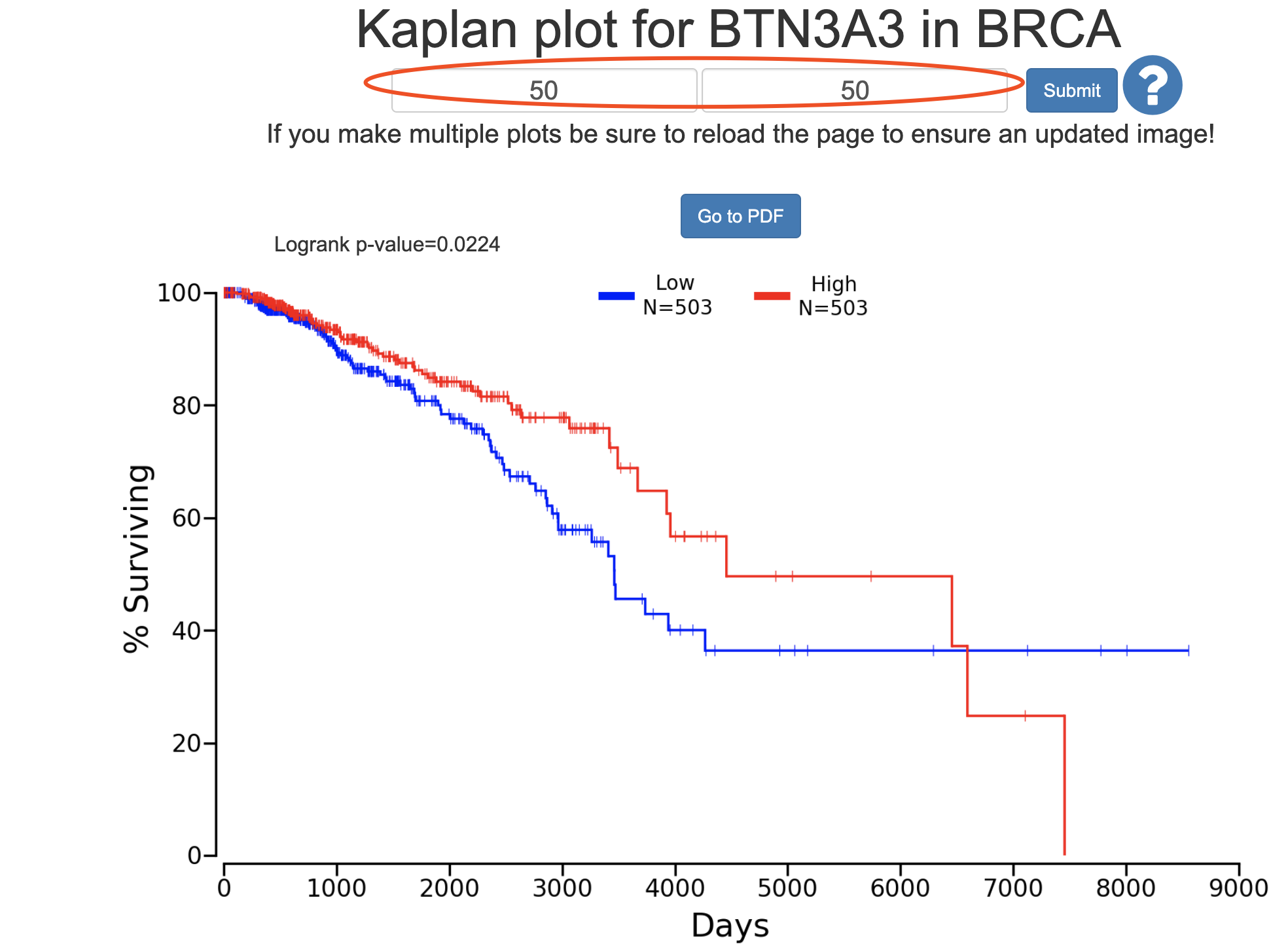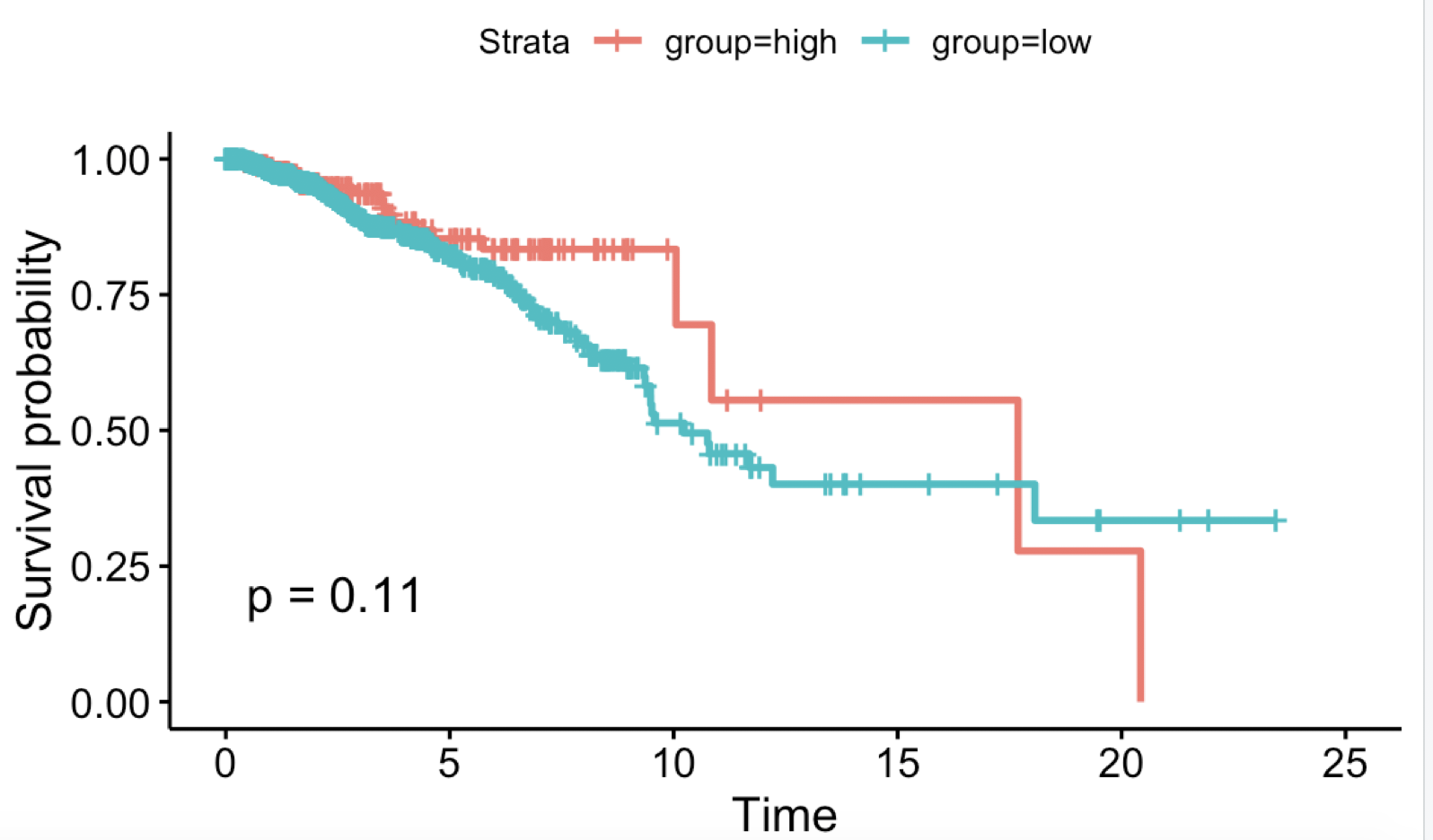# 寻找生存分析的最佳基因表达分组阈值Based on the FPKM value of each gene, we classified the patients into two groups and examined their prognoses.

In the analysis, we excluded genes with low expression, i.e., those with a median expression among samples less than FPKM 1.

The prognosis of each group of patients was examined by Kaplan-Meier survival estimators, and the survival outcomes of the two groups were compared by log-rank tests.

To choose the best FPKM cut-offs for grouping the patients most significantly, all FPKM values from the 20th to 80th percentiles were used to group the patients, significant differences in the survival outcomes of the groups were examined and the value yielding the lowest log-rank P value is selected.``````a=read.csv('BRCA_10384_50_50.csv')
a\$event=ifelse(a\$Status=='Alive',0,1)
library(survival)
library(survminer)
sfit <- survfit(Surv(Days, event)~Group, data=a)
ggsurvplot(sfit, conf.int=F, pval=TRUE)

phe=a
phe\$time=phe\$Days/365

## 批量生存分析 使用 logrank test 方法
mySurv=with(phe,Surv(time, event))
log_rank_p <- lapply(2:8, function(i){
thr=sort(phe\$Expression)[round(nrow(phe)*i/10)]
phe\$group=ifelse(phe\$Expression > thr,'high','low')
print(table( phe\$group ))
data.survdiff=survdiff(mySurv~group,data=phe)
p.val = 1 - pchisq(data.survdiff\$chisq, length(data.survdiff\$n) - 1)
return(p.val)
})
log_rank_p=unlist(log_rank_p)
log_rank_p

i=8
thr=sort(phe\$Expression)[round(nrow(phe)*i/10)]
phe\$group=ifelse(phe\$Expression > thr,'high','low')
print(table( phe\$group ))
sfit <- survfit(Surv(time, event)~group, data=phe)
ggsurvplot(sfit, conf.int=F, pval=TRUE)
``````